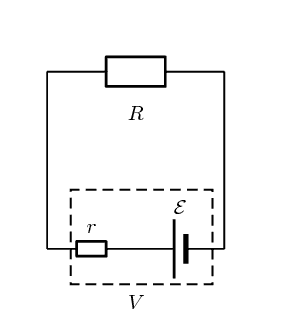# Equation for the Internal Resistance of a Battery

MrNewton
Hello,

i am currently working on a project where i have to calculate the internal resistance of a battery based on the voltage drop caused by placing a resistor over the battery. I am an EE, and i understand how i can calculate the IR by schematic, but i found this equation:

((Open circuit voltage / load circuit voltage) -1) * R 

So the Open circuit voltage (OCV) is the voltage of the battery when nothing is connected to it. The load circuit voltage (LCV) is the voltage on the battery when a resistor (R) is placed.

So let's say OCV = 3.2V
LCD = 2.8V
R = 100 Ohm

So UR = 2.8V
IR = UR/R = 2.8/100 = 0.028A
Ur = OCV-UR = 0.4V

r = Ur/I = 0.4/0.028 = 14.2857 Ohms

If you do this by the first equation you get:
((3.2/2.8)-1)*100 = 14.2857MY QUESTION: How did the get the first equation ? I can't simplify my equations and get the first equation.

Mentor
It's a series resistance, so LCV = R*I = R*OCV/(R+r). Solve for r and you get equation 1.

•vanhees71, cnh1995 and Dale
MrNewton
What? I don't get it. Sorry :D

Gold Member
What? I don't get it. Sorry :D
It's the same as for a simple Potential Divider circuit. The ratio of the voltages across the Load and Internal resistors is the same as the ratio of the resistors. The total voltage is the emf (no load) voltage.
It boils down to solving two simultaneous equations. A bit of lower school Algebra.

•Merlin3189
Mentor
What is unclear?

The total resistance is R+r. This leads to a current of I=OCV/(R+r). The voltage at the outside resistor is then LCV =R*I=OCV/(R+r).
From there it's elementary algebra to get r=... Start by multiplying both sides of equation by (R+r).

Nearly the same steps as you did with numbers, just with the more general variables.

Homework Helper
Gold Member
UR = LCV
i = LCV/R
Ur=OCV-UR
r=Ur/i = (OCV-UR)/i = (OCV-LCV)/i
r=(OCV-LCV)/(LCV/R) = (OCV/LCV -1)R